# Test: Distance Of A Point From A Plane

## 10 Questions MCQ Test Mathematics (Maths) Class 12 | Test: Distance Of A Point From A Plane

Description
This mock test of Test: Distance Of A Point From A Plane for JEE helps you for every JEE entrance exam. This contains 10 Multiple Choice Questions for JEE Test: Distance Of A Point From A Plane (mcq) to study with solutions a complete question bank. The solved questions answers in this Test: Distance Of A Point From A Plane quiz give you a good mix of easy questions and tough questions. JEE students definitely take this Test: Distance Of A Point From A Plane exercise for a better result in the exam. You can find other Test: Distance Of A Point From A Plane extra questions, long questions & short questions for JEE on EduRev as well by searching above.
QUESTION: 1

Solution:
QUESTION: 2

Solution:
QUESTION: 3

### The distance of the point (2, 3, – 5) from the plane x + 2y – 2z = 9 is:​

Solution:

Length of perpendicular from (2,3,-5) to the plane x + 2y − 2z − 9 = 0.
= |(2 + 2×3 −2×(−5) − 9)|√12 + 22 + (−2)2
= |2 + 6 + 10 − 9|/√9
= 9/3
= 3 units.

QUESTION: 4

The angle between the planes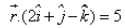and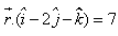is

Solution:
QUESTION: 5

The foot of the perpendicular drawn from the (- 1, – 3, – 5) to a plane is (2, 4, 6). The equation of the plane is:​

Solution:

Since, the foot of the perpendicular to the plane is A(2,4,6). Therefore (4,2,6) is the point on the plane.
So, equation of the plane passing through the point (2,4,6) is:
a(x–2)+b(y–4)+c(z–6)=0.
Now, the direction ratios of the perpendicular line OA=2+1, 4+3, 6+5, i.e., 3,7,11
Therefore, the required plane is:
3(x–2)+7(y–4)+6(z–11)=0
i.e, 3x + 7y + 11z = 100

QUESTION: 6

A point is 5 units away from the vertical plane and 4 units away from profile plane and 3 units away from horizontal plane in 1st quadrant then the projections are drawn on paper the distance between the front view and top view of point is _____________

Solution:

Since the point is 3 units away from the horizontal plane the distance from the point to xy reference line will be 3 units. And then the point is at distance of 5 units from the vertical plane the distance from reference line and point will be 5, sum is 8.

QUESTION: 7

The distance of the plane 6x – 2y + 3z = 12 from the origin is:​

Solution:
QUESTION: 8

The distance of the point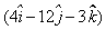from the plane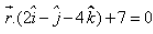is:​

Solution:
QUESTION: 9

Distance of the point P (1, 1, p) from the plane whose equation is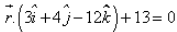is

Solution:
QUESTION: 10

The angle between the line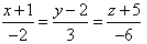and the plane 2x – y + 2z + 7 = 0 is:

Solution:

(x + 1)/(-2) = (y - 2)/(3) = (z + 5)/(-6)
b = -2i + 3j - 6k
n =  2i – yj + 2k
Sinθ = (b.n)/|b||n|
Sinθ = (-2i + 3j - 6k).(2i – yj + 2k)]/{[(-2)2 + (3)2 + (-6)2]^½ *[(2)2 + (-1)2 + (2)2]½}
Sinθ = (-4 -3 -12)/[(49)½ * (9)½]
Sinθ = 19/(7 * 3)
Sinθ= 19/21
θ = sin-1(19/21)